Company News

# How to tell if my robot’s grasp is stable

How to tell if my robot’s grasp is stable

An important part of our roadmap is focusing on making grasping trivial for the end user. We want to be able to point our robot at an object with the instruction of grasp it. Although from a human point of view it sounds trivial, this is actually complicated for a robot. A crucial step in that direction is to be able to quantify how well the robot is grasping the object; without that measurement, the robot will never be able to improve. In this post, we’ll focus on different methods used to assess grasp quality.

We’re adding a few constraints on the grasp quality measurement computation:

• computes in “real time”. We want to be able to use that measurement throughout our grasping pipeline. We also want to display it live to be able to understand what our robot is thinking.
• does not need accurate information about the object. We often do not have much knowledge about the object we want to grasp.
• does not need fine tactile sensing. We want to be able to deploy this on any type of hands. The more tactile data we have the more precise the measurement. But good tactile sensors can be expensive and are not always robust.
• we can use a different metrics to generate grasps.

### Quality measures based on the position of the contact points

The measures we’ll explain below are all based on the same concept. They use the contact points — the points at which the robot is in contact with the object as shown in the figure below — to compute a quality measure for a given grasp.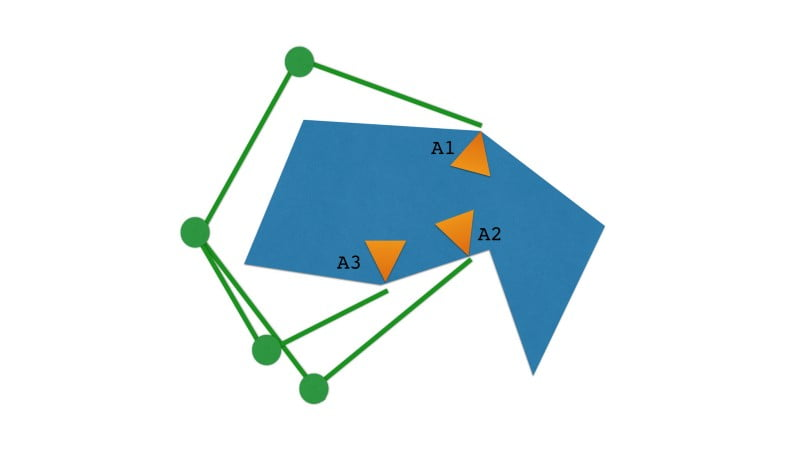#### Area of the grasp polygon

The grasp polygon is the area between the grasping points as can be seen in the figure below. To be able to compute this area, we simply need the points on the hand which are in contact with the object.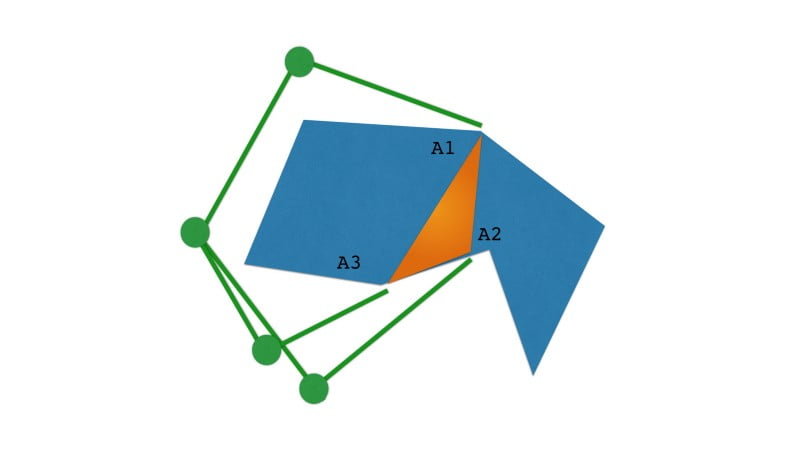This grasp quality measure
can be computed with formula (1). The bigger the grasp polygon, the more stable the grasp. This measurement is quite intuitive: this area is big when the fingers are spread around the biggest part of the object.

It can also be generalised to hands with more than 3 fingers.

#### Shape of the grasp polyhedron

First, what is a polyhedron? It’s the equivalent of a polygone in 3D. So a pyramid, a cube… those are polyhedrons. To make it easier to visualise, we’ll keep using 2D figures — and polygones — in the illustrations below.

This is another approach used to measure the quality of a grasp. Instead of focusing on the volume, we focus on the shape of the polyhedron. Intuitively, we want the fingers to be spread uniformly around the object. Once more we only need the contact points information.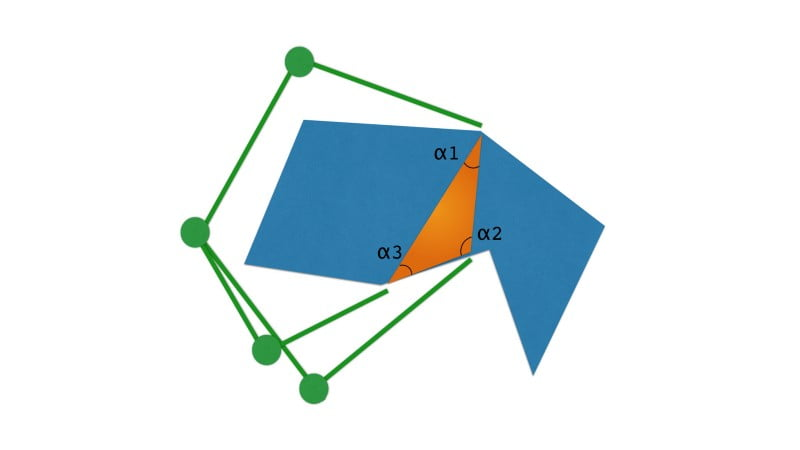This measurement (2) is called the stability grasp index. We are computing the averaged error between the current polyhedron angles and the best configuration, which is the equivalent regular polyhedron. In the example above, our polyhedron — or rather polygone since we’re in 2D — is a triangle. The equivalent regular polyhedron is an equilateral triangle.

where:In this case, the better the grasp, the smaller the grasp measurement.

#### Distance between the center of mass and the center of grasp

This approach focuses on minimising the distance between the center of mass of the object and the center of the grasp polyhedron. It is similar to many algorithms used in legged robot control, where they focus on keeping the projection of the center of mass between the contact points. For this metric, we need to know where the center of mass of the object is. Which means we have to know the pose of the object, as well as some properties of its weight distribution.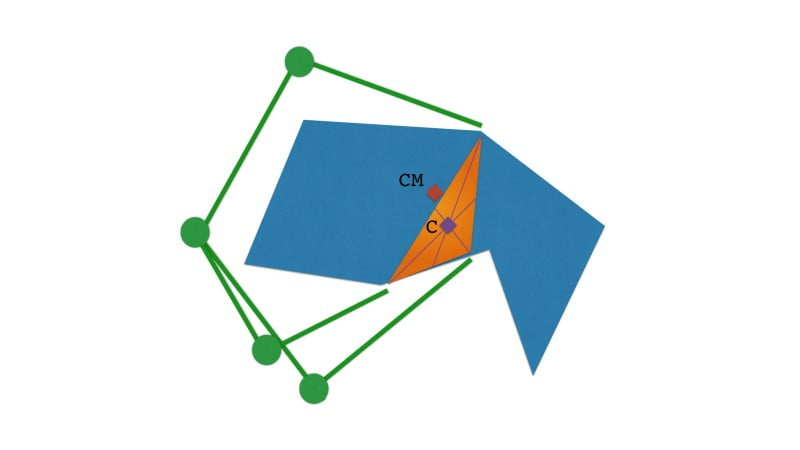What we want here is the centre of the grasp region to be as close as possible to the centre of mass of the object — it’s its equilibrium point. So we want (5) to be as small as possible, the smaller the measure, the more stable the grasp.

### Taking limitations of the finger forces into account

The methods described above all share a common limitation. They do not take into account that the fingers can only apply a limited amount of force.

To solve this limitation, the largest ball criterion was developed. The largest ball criterion is a widely used grasp quality measurement.

The grasp wrench space is easier to represent for a 2D object. It’s a 3D space comprised of the force applied along the x axis, the one around the y axis and the torque around the z axis. As you can see below, you can push that object — in this 2D world — along the x or y axis, or rotate it around it’s z axis. In 3D, the grasp wrench space is a 6D space — translation in x,y,z or rotation around x,y and z.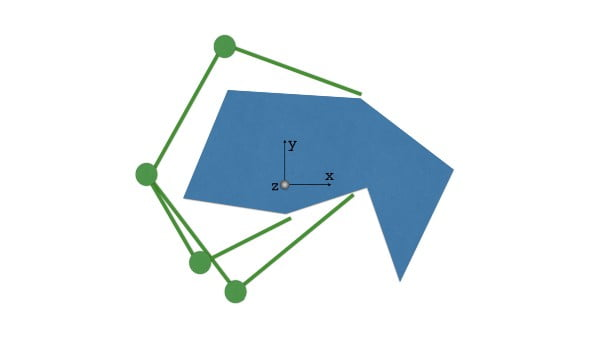We won’t go into the details of how to characterise the grasp wrench space here. But let’s agree that we’re able to compute how much force the hand can apply around the different axis. We just need to know:

• the maximal force each finger can apply
• the possible movements of each joints

So now we’ve drawn our Grasp Wrench Space. This means that we know which external perturbations the robot hand can cope with. If a force applied is outside of the Grasp Wrench Space, then the hand can’t keep the grasp stable. The concept here is that a grasp is only as good as the minimal amount of force applied to the object for the grasp to become unstable. So we want to find which direction of our Grasp Wrench Space is the most fragile. This is the same as finding the distance from the origin to the closest face of the Grasp Wrench Space.

We can solve this problem using geometry. We look for the largest ball centered around the origin of the Grasp Wrench Space while fully contained in that space. You can visualise this in the figure above — on the right.

So the bigger the measure, the more stable the grasp.

### Quality measures based on the hand configuration

These measures rely on the configuration of the hand itself. To simplify, they’re making sure the robot is in as good a position to react as possible. In robotics you often want to avoid being close to singularities. To simplify, when at — or close to — a singularity, a robot can’t move anymore in a given direction.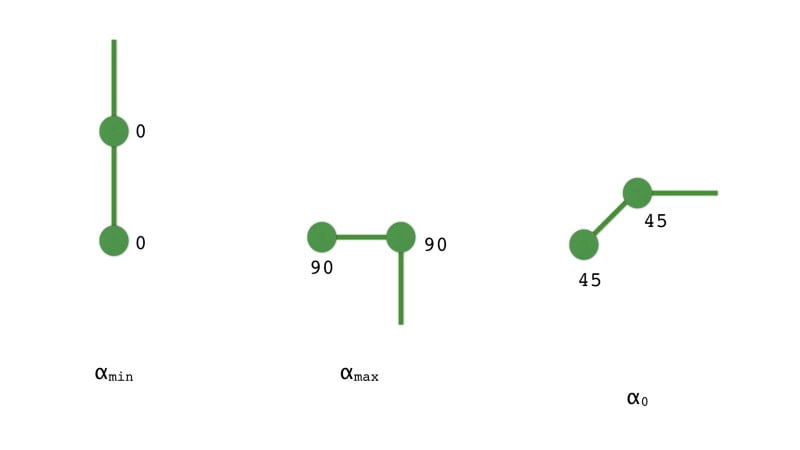To measure this, you can check if each joint of the hand is close to the position in the middle of its range (6). For example for a joint that can go from 0 to 90 degrees, the middle of its range is 45 degrees. So the closer to 45 degrees it is in a given grasp, the easier it is to move it in any direction to counteract an external perturbation. The smaller the measurement, the better the grasp.